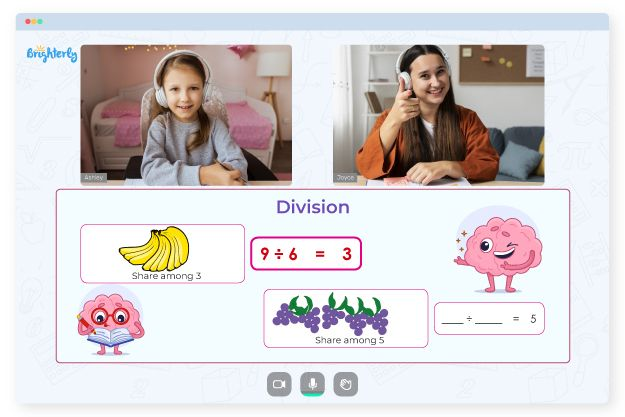# Fractions On A Number Line Worksheets

Math problems that your child will encounter at school require the knowledge of fractions as well as whole numbers. Fractions on a number line worksheet are designed to improve a child’s understanding of fractions, in particular modeling, simplifying, converting, comparing, ordering, and operations. Fractions on number line worksheets are important for developing cognitive arithmetics in a child.

### Why you need fractions on a number line worksheet in PDF

A PDF version of fractions on a number line worksheet will help your child learn this math topic easily. These worksheets are designed to help a child understand the value of fractions and visually identify it. A child can understand how to solve the next fraction in a simple sequence.

With fractions of shapes worksheets, a child can solve fractions of shapes and develop an understanding of fractions as a whole. You can get numerous fraction on the line worksheets in PDF to help a child learn to add fractions on the line, identify fractions, identify mixed numbers, estimate fractions, identify proper fractions, find the missing number on the number line, and more.

Math for Kids

Is Your Child Struggling With Math?
1:1 Online Math Tutoring### Benefits of Fractions on a Number Line Worksheets

These worksheets are useful for teaching students how to plot fractions on a number line. Additionally, a child will form a strong foundation for arithmetic calculations.

Using an equivalent fractions on a number line worksheet, a child can learn basic arithmetic operations such as addition and subtraction. This will boost their problem-solving skills and help them handle other complex problems and real-life issues involving math applications.### Fractions On A Number Line Worksheets PDF

Fractions On A Number Line Worksheet### Fractions On A Number Line Worksheets PDF

Fractions On A Number Line Worksheet PDF### Fractions On A Number Line Worksheets PDF

Equivalent Fractions On A Number Line Worksheet### Fractions On A Number Line Worksheets PDF

Fraction Number Line Worksheets

A child can benefit from a simplified fractions on a number line worksheet in PDF since the sheet contains concise instructions that guide kids and encourage them to use a strategic approach besides keeping children engaged and motivated.

A fractions on a number line worksheet is an effective visual alternative to traditional models like a pizza that are used in explaining fractions. A number on the line worksheet will help a child see a fraction as a number coming between two whole numbers.

### Fractions Worksheets

Need help with Division?• Is your child finding it hard to understand division concepts?
• Try lessons with an online tutor.

Does your child need additional support with division lessons? Start studying with an online tutor.